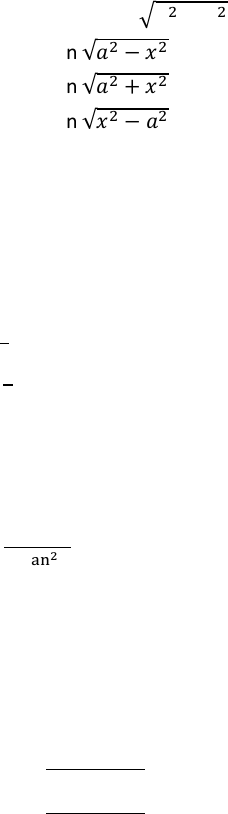Class Notes (1,100,000)
CA (630,000)
UTSG (50,000)
MAT (4,000)
MAT136H1 (900)
all (200)
Lecture

MAT136H1 Lecture Notes - Ellipse, Pythagorean Theorem

Department
Mathematics
Course Code
MAT136H1
Professor
all

Page:
of 17.3 Integration Techniques
Trigonometric Substitution: Overview
Integrals in the form of   are used to find the area of circle or ellipse.
For the expression , the identity      is used for    substitution.
For the expression , the identity      is used for     substitution.
For the expression , the identity     is used for     substitution.
After solving the integral using trigonometric substitutions, substituted variable must be replaced by the
original variable. Based on Pythagorean Theorem, all sides of the right triangle is defined, and THE
substituted variable is replaced by the original variable based on the right triangle.
Half-Angle Formula
 
 

  
Double-Angle Formula
    
          
    

Addition & Subtraction Formula
       
       
        
        
    
   
    
   